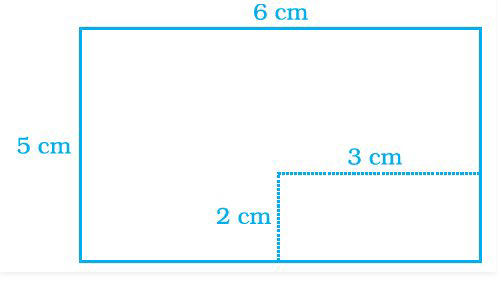# NCERT Exemplar Solutions Class 7 Mathematics Solutions for Perimeter and Area - Exercise in Chapter 9 - Perimeter and Area

A rectangular piece of dimensions 3 cm × 2 cm was cut from a rectangular sheet of paper of dimensions 6 cm × 5 cm (Fig. 9.14).

The area of the remaining sheet of paper is(a) 30 cm2 (b) 36 cm2 (c) 24 cm2 (d) 22 cm2

(c) 24 cm2

First, we have to calculate the area of rectangular piece = length × breadth

= 2 cm × 3 cm

= 6 cm2

So, the area of a sheet of paper of dimensions 6 cm × 5 cm

= 30 cm2

Then, the Area of the remaining sheet of paper is = 30 cm – 6 cm

= 24 cm2

Related Questions
Exercises

Lido

Courses

Teachers

Book a Demo with us

Syllabus

Maths
CBSE
Maths
ICSE
Science
CBSE

Science
ICSE
English
CBSE
English
ICSE
Coding

Terms & Policies

Selina Question Bank

Maths
Physics
Biology

Allied Question Bank

Chemistry
Connect with us on social media!